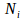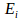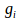## The Boltzmann Distribution

The distribution of the energies of a collection of particles depends on the average energy, which depends on the temperature. The most probable distribution of the energies of a collection of particles depends on the temperature therefore. The Boltzmann distribution expresses this dependence.

The Boltzmann distribution relates the number of particles per state at in terms of the energies of the states:whereis the number of particles at energywhich has degeneracyetc.

Proof: The goal is to findthat maximisesThis expresses the number of ways that a particular distribution of molecules throughout a set of allowed energies can be achieved. Taking logs of this expression, and using Stirling's approximationfor largegivesWe differentiate with respect to generalobtaining(1).

We set this derivative equal to zero. Theare subject to the constraintis fixed, and the energyover and above the zero point energy is also fixed.

Now use Lagrange multipliers to find theis the function(2). We differentiate with respect to eachformingorfor eachSubstitute from (1) to obtainwhich rearranges toWe can eliminate the factorby consideringat two different energies, obtainingConsideration of (2) sincebutfor one degree of freedom so we can writeand further analysis giveshence#### Add commentRefresh